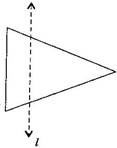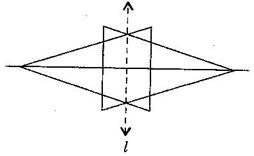### Symmetry - Solutions

CBSE Class –VI Mathematics

NCERT Solutions
Chaper 13 Symmetry (Ex. 13.1)

Question 1. List any four symmetrical objects from your home or school.

Answer: Blackboard, Table top, Notebook, Computer disc

Question 2. For the given figure, which one is the mirror line, ${l}_{1}$ or ${l}_{2}?$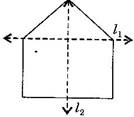Answer: ${l}_{2}$ is the mirror line as both the sides of the lines are symmetric.

Question 3. Identify the shapes given below. Check whether they are symmetric or not. Draw the line of symmetry as well.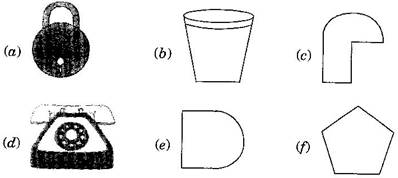(a) Symmetric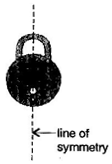(b) Symmetric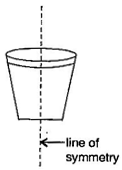(c) Not symmetric

(d) Symmetric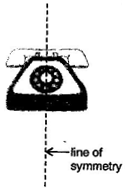(e) Symmetric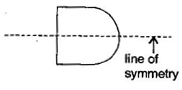(f)Symmetric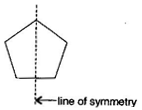Question 4. Copy the following on a square paper. A square paper is what you would have used in your arithmetic notebook in earlier classes. Then complete them such that the dotted line is the line of symmetry.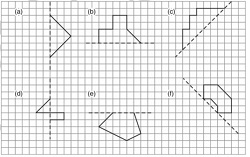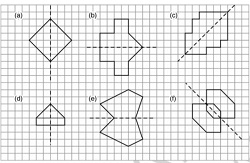Question 5.In the figure, $l$ is the line of symmetry. Complete the diagram to make it symmetric.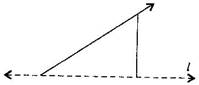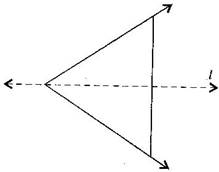Question 6.In the figure, $l$ is the line of symmetry. Draw the image of the triangle and complete the diagram, so that it becomes symmetric.